# Matplotlib Tutorial – A Complete Guide to Python Plot w/ ExamplesThis tutorial explains matplotlib’s way of making plots in simplified parts so you gain the knowledge and a clear understanding of how to build and modify full featured matplotlib plots.

## 1. Introduction

Matplotlib is the most popular plotting library in python. Using matplotlib, you can create pretty much any type of plot. However, as your plots get more complex, the learning curve can get steeper.

The goal of this tutorial is to make you understand ‘how plotting with matplotlib works’ and make you comfortable to build full-featured plots with matplotlib.

## 2. A Basic Scatterplot

The following piece of code is found in pretty much any python code that has matplotlib plots.

import matplotlib.pyplot as plt
%matplotlib inline


matplotlib.pyplot is usually imported as plt. It is the core object that contains the methods to create all sorts of charts and features in a plot.

The %matplotlib inline is a jupyter notebook specific command that let’s you see the plots in the notbook itself.

Suppose you want to draw a specific type of plot, say a scatterplot, the first thing you want to check out are the methods under plt (type plt and hit tab or type dir(plt) in python prompt).

Let’s begin by making a simple but full-featured scatterplot and take it from there. Let’s see what plt.plot() creates if you an arbitrary sequence of numbers.

import matplotlib.pyplot as plt
%matplotlib inline

# Plot
plt.plot([1,2,3,4,10])
#> [<matplotlib.lines.Line2D at 0x10edbab70>]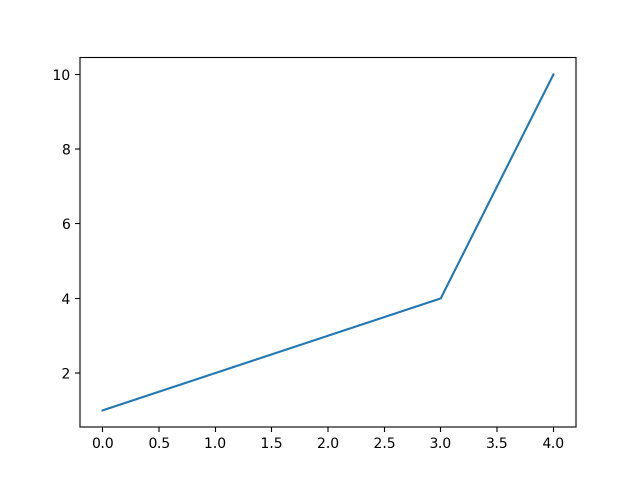I just gave a list of numbers to plt.plot() and it drew a line chart automatically. It assumed the values of the X-axis to start from zero going up to as many items in the data.

Notice the line matplotlib.lines.Line2D in code output?

That’s because Matplotlib returns the plot object itself besides drawing the plot.

If you only want to see the plot, add plt.show() at the end and execute all the lines in one shot.

Alright, notice instead of the intended scatter plot, plt.plot drew a line plot. That’s because of the default behaviour.

So how to draw a scatterplot instead?

Well to do that, let’s understand a bit more about what arguments plt.plot() expects. The plt.plot accepts 3 basic arguments in the following order: (x, y, format).

This format is a short hand combination of {color}{marker}{line}.

For example, the format 'go-' has 3 characters standing for: ‘green colored dots with solid line’. By omitting the line part (‘-‘) in the end, you will be left with only green dots (‘go’), which makes it draw a scatterplot.

Few commonly used short hand format examples are:
* 'r*--' : ‘red stars with dashed lines’
* 'ks.' : ‘black squares with dotted line’ (‘k’ stands for black)
* 'bD-.' : ‘blue diamonds with dash-dot line’.

For a complete list of colors, markers and linestyles, check out the help(plt.plot) command.

Let’s draw a scaterplot with greendots.

# 'go' stands for green dots
plt.plot([1,2,3,4,5], [1,2,3,4,10], 'go')
plt.show()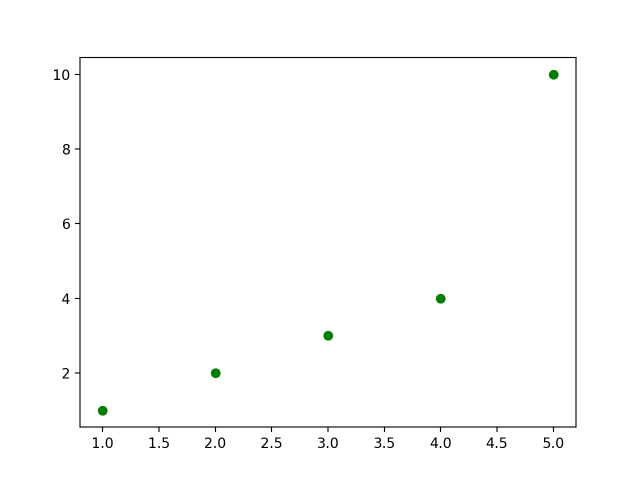## 3. How to draw two sets of scatterplots in same plot

Good. Now how to plot another set of 5 points of different color in the same figure?

Simply call plt.plot() again, it will add those point to the same picture.

You might wonder, why it does not draw these points in a new panel altogether? I will come to that in the next section.

# Draw two sets of points
plt.plot([1,2,3,4,5], [1,2,3,4,10], 'go')  # green dots
plt.plot([1,2,3,4,5], [2,3,4,5,11], 'b*')  # blue stars
plt.show()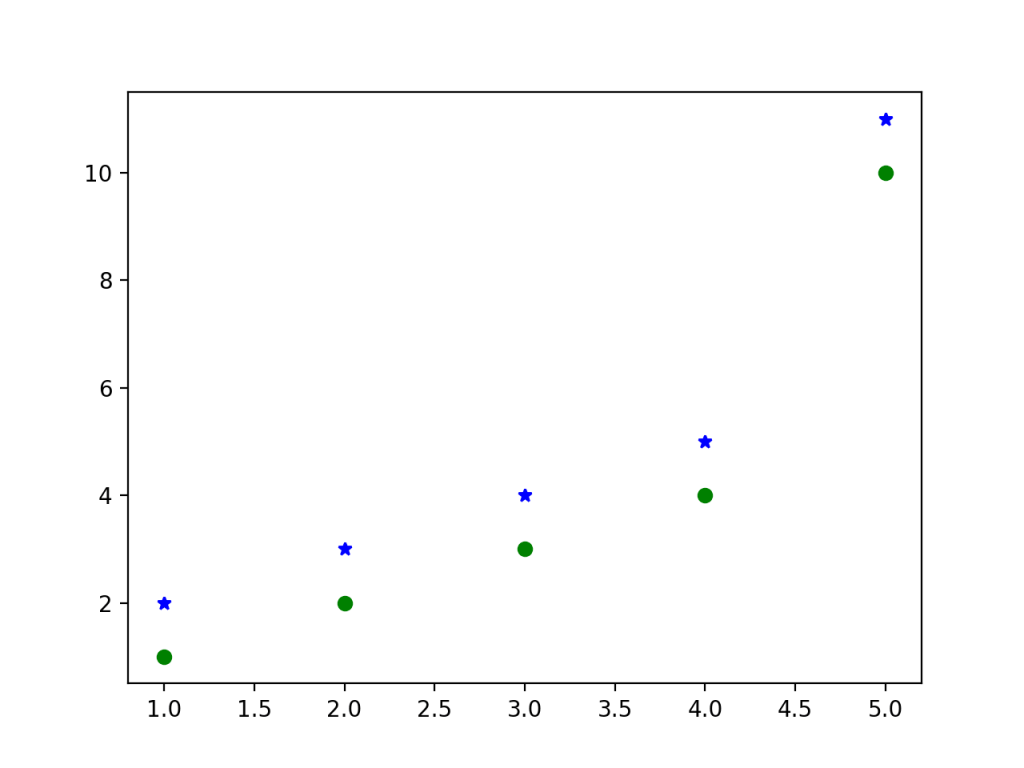Looks good. Now let’s add the basic plot features: Title, Legend, X and Y axis labels. How to do that?

The plt object has corresponding methods to add each of this.

plt.plot([1,2,3,4,5], [1,2,3,4,10], 'go', label='GreenDots')
plt.plot([1,2,3,4,5], [2,3,4,5,11], 'b*', label='Bluestars')
plt.title('A Simple Scatterplot')
plt.xlabel('X')
plt.ylabel('Y')
plt.legend(loc='best')  # legend text comes from the plot's label parameter.
plt.show()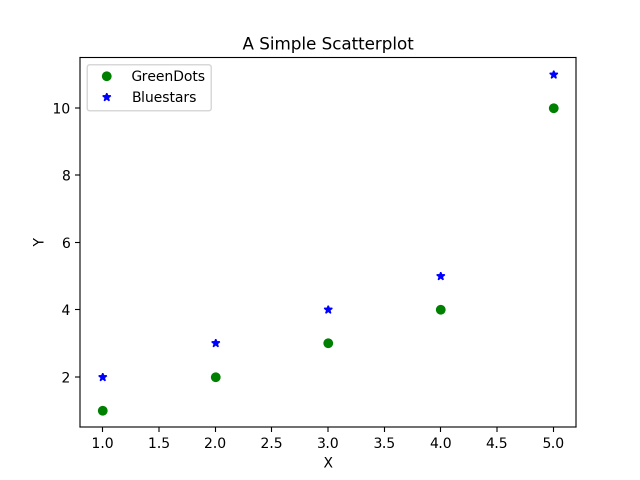Good. Now, how to increase the size of the plot? (The above plot would actually look small on a jupyter notebook)

The easy way to do it is by setting the figsize inside plt.figure() method.

plt.figure(figsize=(10,7)) # 10 is width, 7 is height
plt.plot([1,2,3,4,5], [1,2,3,4,10], 'go', label='GreenDots')  # green dots
plt.plot([1,2,3,4,5], [2,3,4,5,11], 'b*', label='Bluestars')  # blue stars
plt.title('A Simple Scatterplot')
plt.xlabel('X')
plt.ylabel('Y')
plt.xlim(0, 6)
plt.ylim(0, 12)
plt.legend(loc='best')
plt.show()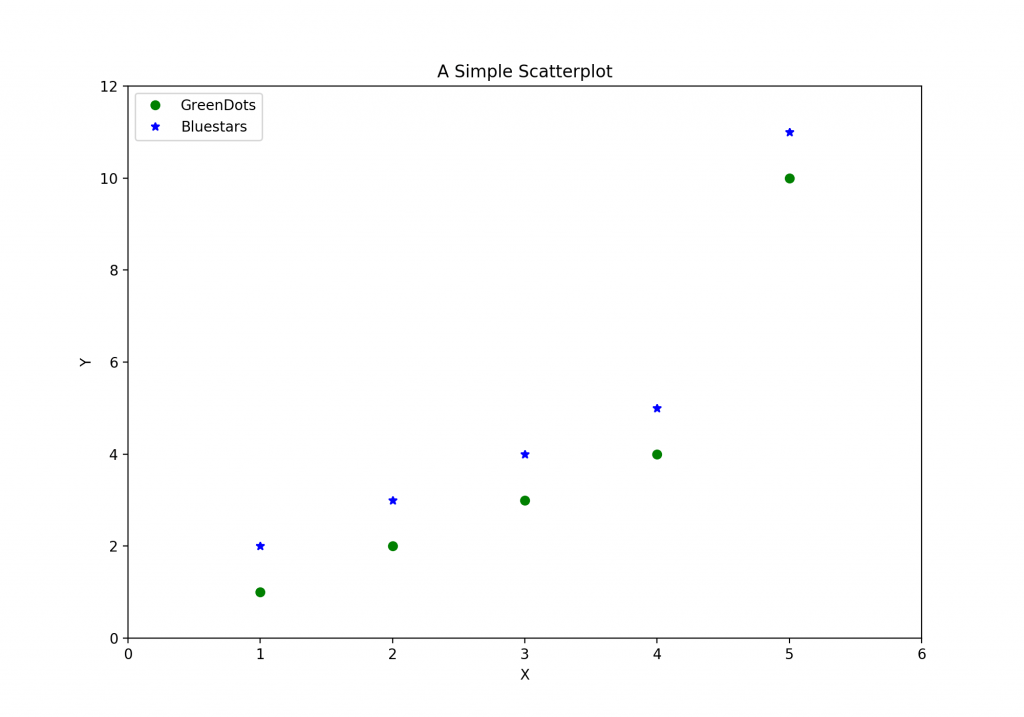Ok, we have some new lines of code there. What does plt.figure do?

Well, every plot that matplotlib makes is drawn on something called 'figure'. You can think of the figure object as a canvas that holds all the subplots and other plot elements inside it.

And a figure can have one or more subplots inside it called axes, arranged in rows and columns. Every figure has atleast one axes. (Don’t confuse this axes with X and Y axis, they are different.)

## 4. How to draw 2 scatterplots in different panels

Let’s understand figure and axes in little more detail.

Suppose, I want to draw our two sets of points (green rounds and blue stars) in two separate plots side-by-side instead of the same plot. How would you do that?

You can do that by creating two separate subplots, aka, axes using plt.subplots(1, 2). This creates and returns two objects:
* the figure
* the axes (subplots) inside the figure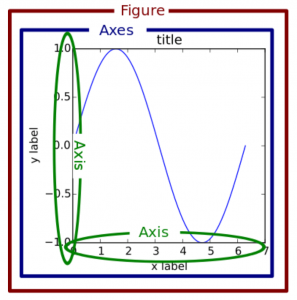Previously, I called plt.plot() to draw the points. Since there was only one axes by default, it drew the points on that axes itself.

But now, since you want the points drawn on different subplots (axes), you have to call the plot function in the respective axes (ax1 and ax2 in below code) instead of plt.

Notice in below code, I call ax1.plot() and ax2.plot() instead of calling plt.plot() twice.

# Create Figure and Subplots
fig, (ax1, ax2) = plt.subplots(1,2, figsize=(10,4), sharey=True, dpi=120)

# Plot
ax1.plot([1,2,3,4,5], [1,2,3,4,10], 'go')  # greendots
ax2.plot([1,2,3,4,5], [2,3,4,5,11], 'b*')  # bluestart

# Title, X and Y labels, X and Y Lim
ax1.set_title('Scatterplot Greendots'); ax2.set_title('Scatterplot Bluestars')
ax1.set_xlabel('X');  ax2.set_xlabel('X')  # x label
ax1.set_ylabel('Y');  ax2.set_ylabel('Y')  # y label
ax1.set_xlim(0, 6) ;  ax2.set_xlim(0, 6)   # x axis limits
ax1.set_ylim(0, 12);  ax2.set_ylim(0, 12)  # y axis limits

# ax2.yaxis.set_ticks_position('none')
plt.tight_layout()
plt.show()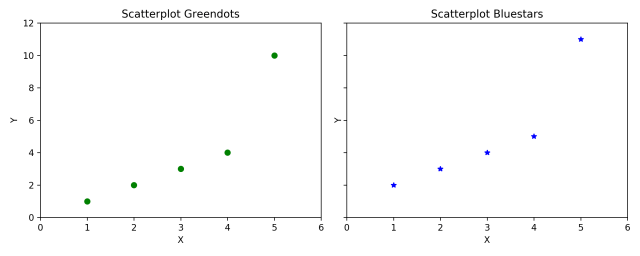Setting sharey=True in plt.subplots() shares the Y axis between the two subplots.

And dpi=120 increased the number of dots per inch of the plot to make it look more sharp and clear. You will notice a distinct improvement in clarity on increasing the dpi especially in jupyter notebooks.

Thats sounds like a lot of functions to learn. Well it’s quite easy to remember it actually.

The ax1 and ax2 objects, like plt, has equivalent set_title, set_xlabel and set_ylabel functions. Infact, the plt.title() actually calls the current axes set_title() to do the job.

• plt.xlabel() → ax.set_xlabel()
• plt.ylabel() → ax.set_ylabel()
• plt.xlim() → ax.set_xlim()
• plt.ylim() → ax.set_ylim()
• plt.title() → ax.set_title()

Alternately, to save keystrokes, you can set multiple things in one go using the ax.set().

ax1.set(title='Scatterplot Greendots', xlabel='X', ylabel='Y', xlim=(0,6), ylim=(0,12))
ax2.set(title='Scatterplot Bluestars', xlabel='X', ylabel='Y', xlim=(0,6), ylim=(0,12))


## 5. Object Oriented Syntax vs Matlab like Syntax

A known ‘problem’ with learning matplotlib is, it has two coding interfaces:

1. Matlab like syntax
2. Object oriented syntax.

This is partly the reason why matplotlib doesn’t have one consistent way of achieving the same given output, making it a bit difficult to understand for new comers.

The syntax you’ve seen so far is the Object-oriented syntax, which I personally prefer and is more intuitive and pythonic to work with.

However, since the original purpose of matplotlib was to recreate the plotting facilities of matlab in python, the matlab-like-syntax is retained and still works.

The matlab syntax is ‘stateful’.

That means, the plt keeps track of what the current axes is. So whatever you draw with plt.{anything} will reflect only on the current subplot.

Practically speaking, the main difference between the two syntaxes is, in matlab-like syntax, all plotting is done using plt methods instead of the respective axes‘s method as in object oriented syntax.

So, how to recreate the above multi-subplots figure (or any other figure for that matter) using matlab-like syntax?

The general procedure is: You manually create one subplot at a time (using plt.subplot() or plt.add_subplot()) and immediately call plt.plot() or plt.{anything} to modify that specific subplot (axes). Whatever method you call using plt will be drawn in the current axes.

The code below shows this in practice.

plt.figure(figsize=(10,4), dpi=120) # 10 is width, 4 is height

# Left hand side plot
plt.subplot(1,2,1)  # (nRows, nColumns, axes number to plot)
plt.plot([1,2,3,4,5], [1,2,3,4,10], 'go')  # green dots
plt.title('Scatterplot Greendots')
plt.xlabel('X'); plt.ylabel('Y')
plt.xlim(0, 6); plt.ylim(0, 12)

# Right hand side plot
plt.subplot(1,2,2)
plt.plot([1,2,3,4,5], [2,3,4,5,11], 'b*')  # blue stars
plt.title('Scatterplot Bluestars')
plt.xlabel('X'); plt.ylabel('Y')
plt.xlim(0, 6); plt.ylim(0, 12)
plt.show()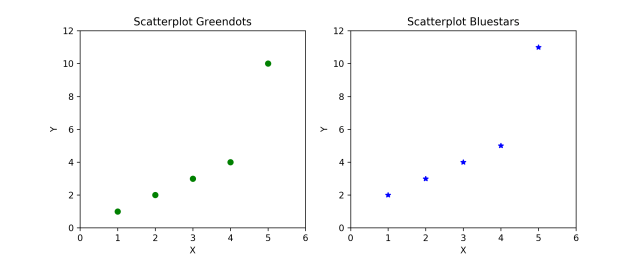Let’s breakdown the above piece of code.

In plt.subplot(1,2,1), the first two values, that is (1,2) specifies the number of rows (1) and columns (2) and the third parameter (1) specifies the position of current subplot. The subsequent plt functions, will always draw on this current subplot.

You can get a reference to the current (subplot) axes with plt.gca() and the current figure with plt.gcf(). Likewise, plt.cla() and plt.clf() will clear the current axes and figure respectively.

Alright, compare the above code with the object oriented (OO) version. The OO version might look a but confusing because it has a mix of both ax1 and plt commands.

However, there is a significant advantage with axes approach.

That is, since plt.subplots returns all the axes as separate objects, you can avoid writing repetitive code by looping through the axes.

Always remember: plt.plot() or plt.{anything} will always act on the plot in the current axes, whereas, ax.{anything} will modify the plot inside that specific ax.

# Draw multiple plots using for-loops using object oriented syntax
import numpy as np
from numpy.random import seed, randint
seed(100)

# Create Figure and Subplots
fig, axes = plt.subplots(2,2, figsize=(10,6), sharex=True, sharey=True, dpi=120)

# Define the colors and markers to use
colors = {0:'g', 1:'b', 2:'r', 3:'y'}
markers = {0:'o', 1:'x', 2:'*', 3:'p'}

# Plot each axes
for i, ax in enumerate(axes.ravel()):
ax.plot(sorted(randint(0,10,10)), sorted(randint(0,10,10)), marker=markers[i], color=colors[i])
ax.set_title('Ax: ' + str(i))
ax.yaxis.set_ticks_position('none')

plt.suptitle('Four Subplots in One Figure', verticalalignment='bottom', fontsize=16)
plt.tight_layout()
plt.show()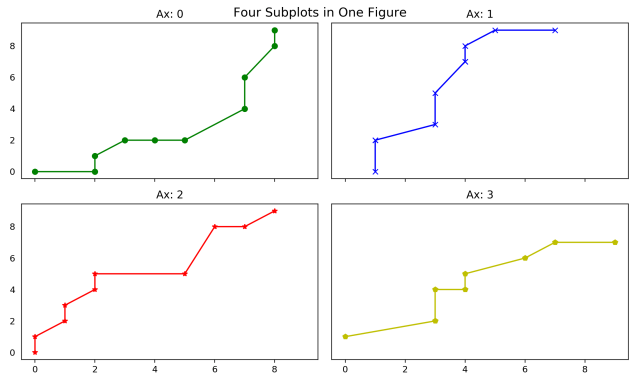Did you notice in above plot, the Y-axis does not have ticks?

That’s because I used ax.yaxis.set_ticks_position('none') to turn off the Y-axis ticks. This is another advantage of the object-oriented interface. You can actually get a reference to any specific element of the plot and use its methods to manipulate it.

Can you guess how to turn off the X-axis ticks?

The plt.suptitle() added a main title at figure level title. plt.title() would have done the same for the current subplot (axes).

The verticalalignment='bottom' parameter denotes the hingepoint should be at the bottom of the title text, so that the main title is pushed slightly upwards.

Alright, What you’ve learned so far is the core essence of how to create a plot and manipulate it using matplotlib. Next, let’s see how to get the reference to and modify the other components of the plot

## 6. How to Modify the Axis Ticks Positions and Labels

There are 3 basic things you will probably ever need in matplotlib when it comes to manipulating axis ticks:
1. How to control the position and tick labels? (using plt.xticks() or ax.setxticks() and ax.setxticklabels())
2. How to control which axis’s ticks (top/bottom/left/right) should be displayed (using plt.tick_params())
3. Functional formatting of tick labels

If you are using ax syntax, you can use ax.set_xticks() and ax.set_xticklabels() to set the positions and label texts respectively. If you are using the plt syntax, you can set both the positions as well as the label text in one call using the plt.xticks().

Actually, if you look at the code of plt.xticks() method (by typing ??plt.xticks in jupyter notebook), it calls ax.set_xticks() and ax.set_xticklabels() to do the job. plt.xticks takes the ticks and labels as required parameters but you can also adjust the label’s fontsize, rotation, ‘horizontalalignment’ and ‘verticalalignment’ of the hinge points on the labels, like I’ve done in the below example.

from matplotlib.ticker import FuncFormatter

return round(x * 57.2985, 2)

plt.figure(figsize=(12,7), dpi=100)
X = np.linspace(0,2*np.pi,1000)
plt.plot(X,np.sin(X))
plt.plot(X,np.cos(X))

# 1. Adjust x axis Ticks
plt.xticks(ticks=np.arange(0, 440/57.2985, 90/57.2985), fontsize=12, rotation=30, ha='center', va='top')  # 1 radian = 57.2985 degrees

# 2. Tick Parameters
plt.tick_params(axis='both',bottom=True, top=True, left=True, right=True, direction='in', which='major', grid_color='blue')

# 3. Format tick labels to convert radians to degrees
plt.gca().xaxis.set_major_formatter(formatter)

plt.grid(linestyle='--', linewidth=0.5, alpha=0.15)
plt.title('Sine and Cosine Waves\n(Notice the ticks are on all 4 sides pointing inwards, radians converted to degrees in x axis)', fontsize=14)
plt.show()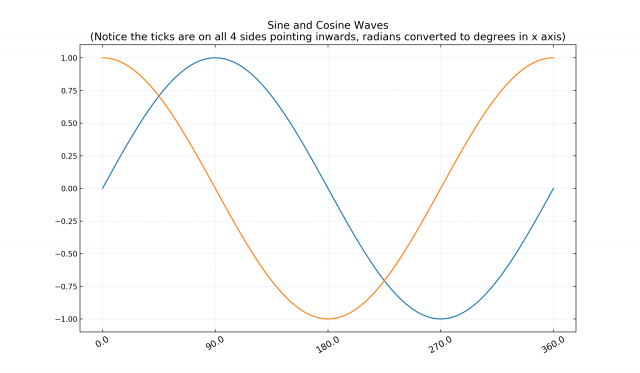In above code, plt.tick_params() is used to determine which all axis of the plot (‘top’ / ‘bottom’ / ‘left’ / ‘right’) you want to draw the ticks and which direction (‘in’ / ‘out’) the tick should point to.

the matplotlib.ticker module provides the FuncFormatter to determine how the final tick label should be shown.

## 7. Understanding the rcParams, Colors and Plot Styles

The look and feel of various components of a matplotlib plot can be set globally using rcParams. The complete list of rcParams can be viewed by typing:

mpl.rc_params()
# RcParams({'_internal.classic_mode': False,
#           'agg.path.chunksize': 0,
#           'animation.avconv_args': [],
#           'animation.avconv_path': 'avconv',
#           'animation.bitrate': -1,
#           'animation.codec': 'h264',
#           ... TRUNCATED LaRge OuTPut ...


You can adjust the params you’d like to change by updating it. The below snippet adjusts the font by setting it to ‘stix’, which looks great on plots by the way.

mpl.rcParams.update({'font.size': 18, 'font.family': 'STIXGeneral', 'mathtext.fontset': 'stix'})


After modifying a plot, you can rollback the rcParams to default setting using:

mpl.rcParams.update(mpl.rcParamsDefault)  # reset to defaults


Matplotlib comes with pre-built styles which you can look by typing:

plt.style.available
# ['seaborn-dark', 'seaborn-darkgrid', 'seaborn-ticks', 'fivethirtyeight',
#  'seaborn-whitegrid', 'classic', '_classic_test', 'fast', 'seaborn-talk',
#  'seaborn-dark-palette', 'seaborn-bright', 'seaborn-pastel', 'grayscale',
#  'seaborn-notebook', 'ggplot', 'seaborn-colorblind', 'seaborn-muted',
#  'seaborn', 'Solarize_Light2', 'seaborn-paper', 'bmh', 'tableau-colorblind10',
#  'seaborn-white', 'dark_background', 'seaborn-poster', 'seaborn-deep']

import matplotlib as mpl
mpl.rcParams.update({'font.size': 18, 'font.family': 'STIXGeneral', 'mathtext.fontset': 'stix'})

def plot_sine_cosine_wave(style='ggplot'):
plt.style.use(style)
plt.figure(figsize=(7,4), dpi=80)
X = np.linspace(0,2*np.pi,1000)
plt.plot(X,np.sin(X)); plt.plot(X,np.cos(X))
plt.xticks(ticks=np.arange(0, 440/57.2985, 90/57.2985), labels = [r'$0$',r'$\frac{\pi}{2}$',r'$\pi$',r'$\frac{3\pi}{2}$',r'$2\pi$'])  # 1 radian = 57.2985 degrees
plt.gca().set(ylim=(-1.25, 1.25), xlim=(-.5, 7))
plt.title(style, fontsize=18)
plt.show()

plot_sine_cosine_wave('seaborn-notebook')
plot_sine_cosine_wave('ggplot')
plot_sine_cosine_wave('bmh')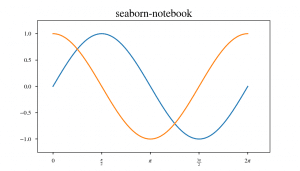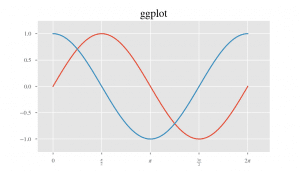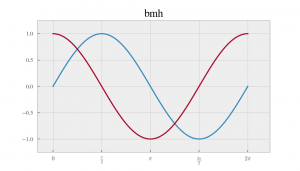I’ve just shown few of the pre-built styles, the rest of the list is definitely worth a look.

Matplotlib also comes with pre-built colors and palettes. Type the following in your jupyter/python console to check out the available colors.

# View Colors
mpl.colors.CSS4_COLORS  # 148 colors
mpl.colors.XKCD_COLORS  # 949 colors
mpl.colors.BASE_COLORS  # 8 colors
#> {'b': (0, 0, 1),
#>  'g': (0, 0.5, 0),
#>  'r': (1, 0, 0),
#>  'c': (0, 0.75, 0.75),
#>  'm': (0.75, 0, 0.75),
#>  'y': (0.75, 0.75, 0),
#>  'k': (0, 0, 0),
#>  'w': (1, 1, 1)}

# View first 10 Palettes
dir(plt.cm)[:10]
#> ['Accent', 'Accent_r', 'Blues', 'Blues_r',
#>  'BrBG', 'BrBG_r', 'BuGn', 'BuGn_r', 'BuPu', 'BuPu_r']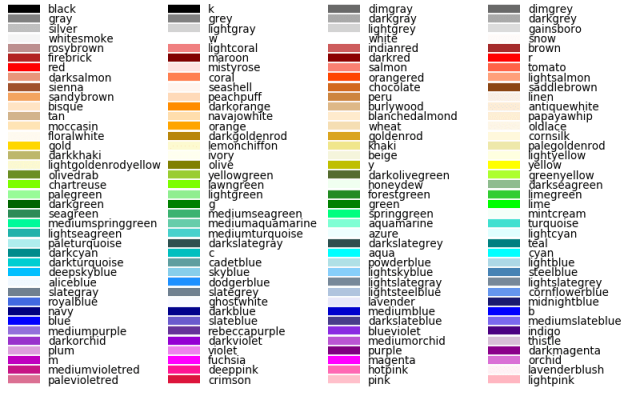## 8. How to Customise the Legend

The most common way to make a legend is to define the label parameter for each of the plots and finally call plt.legend().

However, sometimes you might want to construct the legend on your own. In that case, you need to pass the plot items you want to draw the legend for and the legend text as parameters to plt.legend() in the following format:

plt.legend((line1, line2, line3), ('label1', 'label2', 'label3'))

# plt.style.use('seaborn-notebook')
plt.figure(figsize=(10,7), dpi=80)
X = np.linspace(0, 2*np.pi, 1000)
sine = plt.plot(X,np.sin(X)); cosine = plt.plot(X,np.cos(X))
sine_2 = plt.plot(X,np.sin(X+.5)); cosine_2 = plt.plot(X,np.cos(X+.5))
plt.gca().set(ylim=(-1.25, 1.5), xlim=(-.5, 7))
plt.title('Custom Legend Example', fontsize=18)

# Modify legend
plt.legend([sine, cosine, sine_2, cosine_2],   # plot items
['sine curve', 'cosine curve', 'sine curve 2', 'cosine curve 2'],
frameon=True,                                   # legend border
framealpha=1,                                   # transparency of border
ncol=2,                                         # num columns
title='Sines and Cosines')                      # title
plt.show()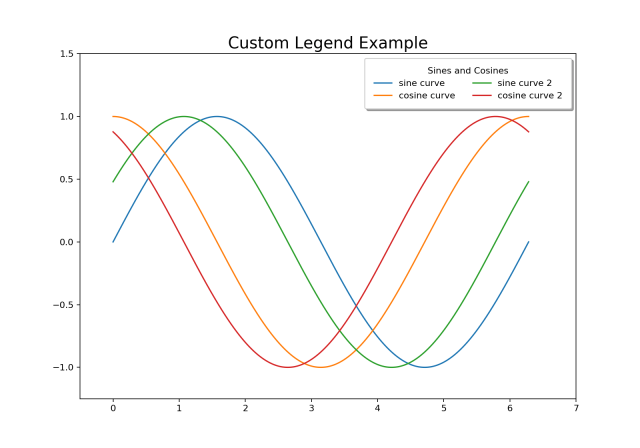## 9. How to Add Texts, Arrows and Annotations

plt.text and plt.annotate adds the texts and annotations respectively. If you have to plot multiple texts you need to call plt.text() as many times typically in a for-loop.

Let’s annotate the peaks and troughs adding arrowprops and a bbox for the text.

# Texts, Arrows and Annotations Example
# ref: https://matplotlib.org/users/annotations_guide.html
plt.figure(figsize=(14,7), dpi=120)
X = np.linspace(0, 8*np.pi, 1000)
sine = plt.plot(X,np.sin(X), color='tab:blue');

# 1. Annotate with Arrow Props and bbox
plt.annotate('Peaks', xy=(90/57.2985, 1.0), xytext=(90/57.2985, 1.5),
bbox=dict(boxstyle='square', fc='green', linewidth=0.1),
arrowprops=dict(facecolor='green', shrink=0.01, width=0.1),
fontsize=12, color='white', horizontalalignment='center')

# 2. Texts at Peaks and Troughs
for angle in [440, 810, 1170]:
plt.text(angle/57.2985, 1.05, str(angle) + "\ndegrees", transform=plt.gca().transData, horizontalalignment='center', color='green')

for angle in [270, 630, 990, 1350]:
plt.text(angle/57.2985, -1.3, str(angle) + "\ndegrees", transform=plt.gca().transData, horizontalalignment='center', color='red')

plt.gca().set(ylim=(-2.0, 2.0), xlim=(-.5, 26))
plt.title('Annotations and Texts Example', fontsize=18)
plt.show()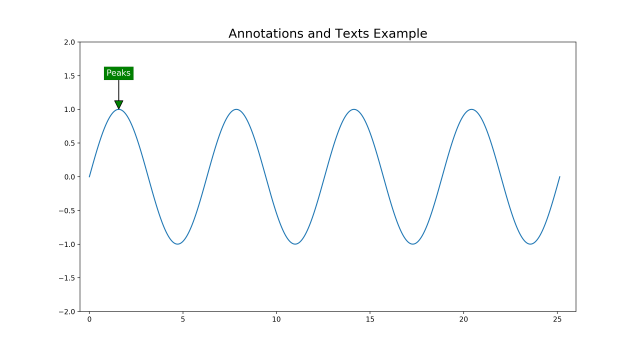Notice, all the text we plotted above was in relation to the data.

That is, the x and y position in the plt.text() corresponds to the values along the x and y axes. However, sometimes you might work with data of different scales on different subplots and you want to write the texts in the same position on all the subplots.

In such case, instead of manually computing the x and y positions for each axes, you can specify the x and y values in relation to the axes (instead of x and y axis values).

You can do this by setting transform=ax.transData.

The lower left corner of the axes has (x,y) = (0,0) and the top right corner will correspond to (1,1).

The below plot shows the position of texts for the same values of (x,y) = (0.50, 0.02) with respect to the Data(transData), Axes(transAxes) and Figure(transFigure) respectively.

# Texts, Arrows and Annotations Example
plt.figure(figsize=(14,7), dpi=80)
X = np.linspace(0, 8*np.pi, 1000)

# Text Relative to DATA
plt.text(0.50, 0.02, "Text relative to the DATA centered at : (0.50, 0.02)", transform=plt.gca().transData, fontsize=14, ha='center', color='blue')

# Text Relative to AXES
plt.text(0.50, 0.02, "Text relative to the AXES centered at : (0.50, 0.02)", transform=plt.gca().transAxes, fontsize=14, ha='center', color='blue')

# Text Relative to FIGURE
plt.text(0.50, 0.02, "Text relative to the FIGURE centered at : (0.50, 0.02)", transform=plt.gcf().transFigure, fontsize=14, ha='center', color='blue')

plt.gca().set(ylim=(-2.0, 2.0), xlim=(0, 2))
plt.title('Placing Texts Relative to Data, Axes and Figure', fontsize=18)
plt.show()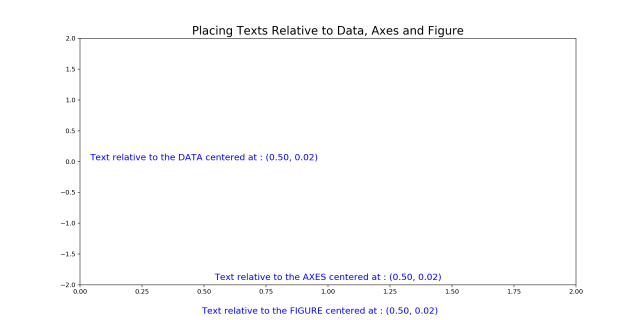## 10. How to customize matplotlib’s subplots layout

Matplotlib provides two convenient ways to create customized multi-subplots layout.

• plt.subplot2grid
• plt.GridSpec

Both plt.subplot2grid and plt.GridSpec lets you draw complex layouts. Below is a nice plt.subplot2grid example.

# Supplot2grid approach
fig = plt.figure()
ax1 = plt.subplot2grid((3,3), (0,0), colspan=2, rowspan=2) # topleft
ax3 = plt.subplot2grid((3,3), (0,2), rowspan=3)            # right
ax4 = plt.subplot2grid((3,3), (2,0))                       # bottom left
ax5 = plt.subplot2grid((3,3), (2,1))                       # bottom right
fig.tight_layout()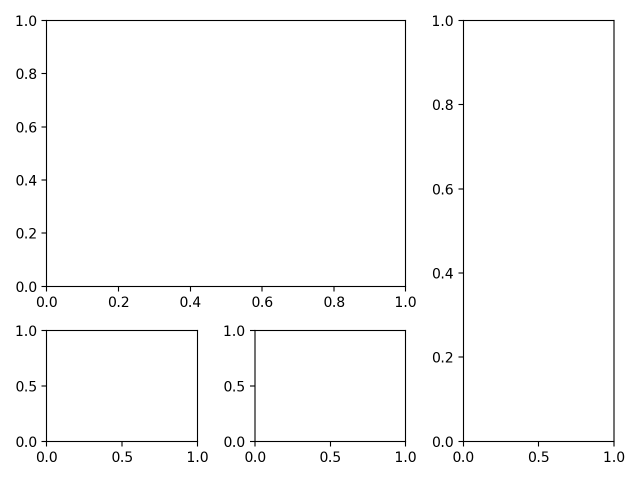Using plt.GridSpec, you can use either a plt.subplot() interface which takes part of the grid specified by plt.GridSpec(nrow, ncol) or use the ax = fig.add_subplot(g) where the GridSpec is defined by height_ratios and weight_ratios.

# GridSpec Approach 1
import matplotlib.gridspec as gridspec
fig = plt.figure()
grid = plt.GridSpec(2, 3)  # 2 rows 3 cols
plt.subplot(grid[0, :2])  # top left
plt.subplot(grid[0, 2])   # top right
plt.subplot(grid[1, :1])  # bottom left
plt.subplot(grid[1, 1:])  # bottom right
fig.tight_layout()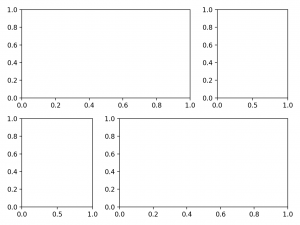# GridSpec Approach 2
import matplotlib.gridspec as gridspec
fig = plt.figure()
gs = gridspec.GridSpec(2, 2, height_ratios=[2,1], width_ratios=[1,2])
for g in gs:
fig.tight_layout()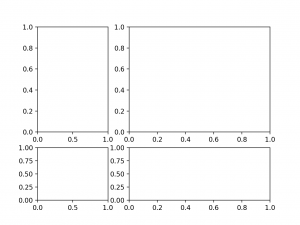The above examples showed layouts where the subplots dont overlap. It is possible to make subplots to overlap. Infact you can draw an axes inside a larger axes using fig.add_axes(). You need to specify the x,y positions relative to the figure and also the width and height of the inner plot.

Below is an example of an inner plot that zooms in to a larger plot.

# Plot inside a plot
plt.style.use('seaborn-whitegrid')
fig, ax = plt.subplots(figsize=(10,6))
x = np.linspace(-0.50, 1., 1000)

# Outer Plot
ax.plot(x, x**2)
ax.plot(x, np.sin(x))
ax.set(xlim=(-0.5, 1.0), ylim=(-0.5,1.2))
fig.tight_layout()

# Inner Plot
inner_ax = fig.add_axes([0.2, 0.55, 0.35, 0.35]) # x, y, width, height
inner_ax.plot(x, x**2)
inner_ax.plot(x, np.sin(x))
inner_ax.set(title='Zoom In', xlim=(-.2, .2), ylim=(-.01, .02),
yticks = [-0.01, 0, 0.01, 0.02], xticks=[-0.1,0,.1])
ax.set_title("Plot inside a Plot", fontsize=20)
plt.show()
mpl.rcParams.update(mpl.rcParamsDefault)  # reset to defaults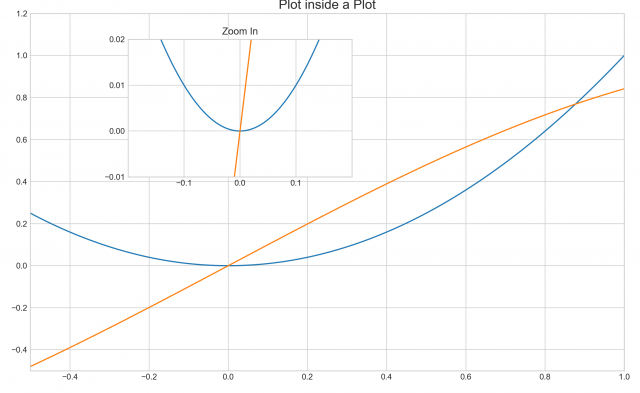## 11. How is scatterplot drawn with plt.plot different from plt.scatter

The difference is plt.plot() does not provide options to change the color and size of point dynamically (based on another array). But plt.scatter() allows you to do that.

By varying the size and color of points, you can create nice looking bubble plots.

Another convenience is you can directly use a pandas dataframe to set the x and y values, provided you specify the source dataframe in the data argument.

You can also set the color 'c' and size 's' of the points from one of the dataframe columns itself.

# Scatterplot with varying size and color of points
import pandas as pd

# Plot
fig = plt.figure(figsize=(14, 7), dpi= 80, facecolor='w', edgecolor='k')
plt.scatter('area', 'poptotal', data=midwest, s='dot_size', c='popdensity', cmap='Reds', edgecolors='black', linewidths=.5)
plt.title("Bubble Plot of PopTotal vs Area\n(color: 'popdensity' & size: 'dot_size' - both are numeric columns in midwest)", fontsize=16)
plt.xlabel('Area', fontsize=18)
plt.ylabel('Poptotal', fontsize=18)
plt.colorbar()
plt.show()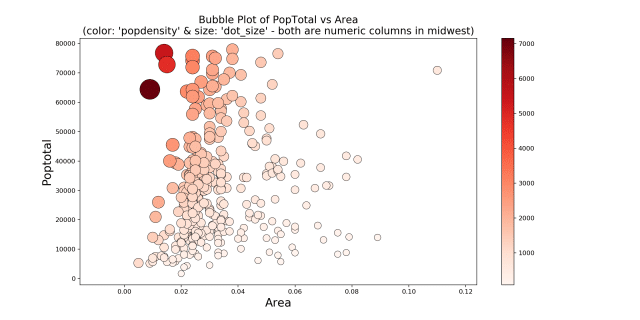# Import data
import pandas as pd

# Plot
fig = plt.figure(figsize=(14, 9), dpi= 80, facecolor='w', edgecolor='k')
colors = plt.cm.tab20.colors
categories = np.unique(midwest['category'])
for i, category in enumerate(categories):
plt.scatter('area', 'poptotal', data=midwest.loc[midwest.category==category, :], s='dot_size', c=colors[i], label=str(category), edgecolors='black', linewidths=.5)

# Legend for size of points
for dot_size in [100, 300, 1000]:
plt.scatter([], [], c='k', alpha=0.5, s=dot_size, label=str(dot_size) + ' TotalPop')
plt.legend(loc='upper right', scatterpoints=1, frameon=False, labelspacing=2, title='Saravana Stores', fontsize=8)
plt.title("Bubble Plot of PopTotal vs Area\n(color: 'category' - a categorical column in midwest)", fontsize=18)
plt.xlabel('Area', fontsize=16)
plt.ylabel('Poptotal', fontsize=16)
plt.show()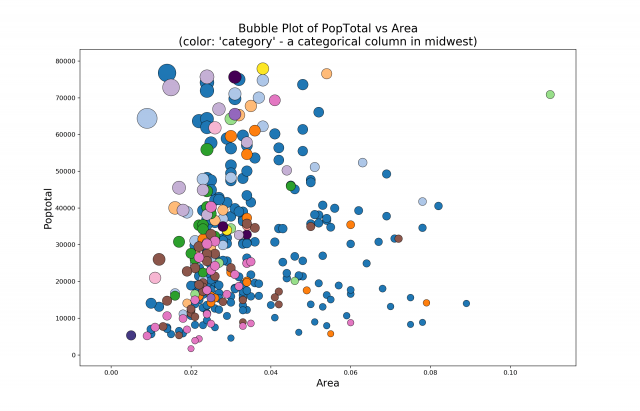# Save the figure
plt.savefig("bubbleplot.png", transparent=True, dpi=120)


## 12. How to draw Histograms, Boxplots and Time Series

The methods to draw different types of plots are present in pyplot (plt) as well as Axes. The below example shows basic examples of few of the commonly used plot types.

import pandas as pd

# Setup the subplot2grid Layout
fig = plt.figure(figsize=(10, 5))
ax1 = plt.subplot2grid((2,4), (0,0))
ax2 = plt.subplot2grid((2,4), (0,1))
ax3 = plt.subplot2grid((2,4), (0,2))
ax4 = plt.subplot2grid((2,4), (0,3))
ax5 = plt.subplot2grid((2,4), (1,0), colspan=2)
ax6 = plt.subplot2grid((2,4), (1,2))
ax7 = plt.subplot2grid((2,4), (1,3))

# Input Arrays
n = np.array([0,1,2,3,4,5])
x = np.linspace(0,5,10)
xx = np.linspace(-0.75, 1., 100)

# Scatterplot
ax1.scatter(xx, xx + np.random.randn(len(xx)))
ax1.set_title("Scatter Plot")

# Step Chart
ax2.step(n, n**2, lw=2)
ax2.set_title("Step Plot")

# Bar Chart
ax3.bar(n, n**2, align="center", width=0.5, alpha=0.5)
ax3.set_title("Bar Chart")

# Fill Between
ax4.fill_between(x, x**2, x**3, color="steelblue", alpha=0.5);
ax4.set_title("Fill Between");

# Time Series
dates = pd.date_range('2018-01-01', periods = len(xx))
ax5.plot(dates, xx + np.random.randn(len(xx)))
ax5.set_xticks(dates[::30])
ax5.set_xticklabels(dates.strftime('%Y-%m-%d')[::30])
ax5.set_title("Time Series")

# Box Plot
ax6.boxplot(np.random.randn(len(xx)))
ax6.set_title("Box Plot")

# Histogram
ax7.hist(xx + np.random.randn(len(xx)))
ax7.set_title("Histogram")

fig.tight_layout()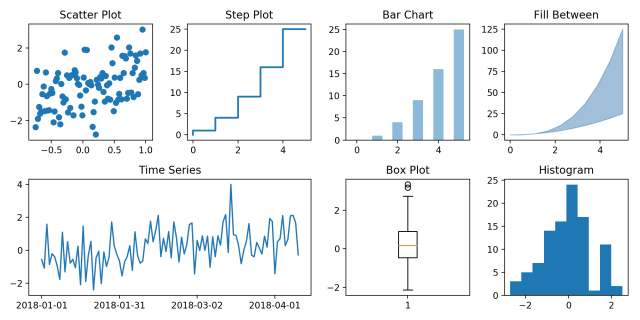If you want to see more data analysis oriented examples of a particular plot type, say histogram or time series, the top 50 master plots for data analysis will give you concrete examples of presentation ready plots. This is a very useful tool to have, not only to construct nice looking plots but to draw ideas to what type of plot you want to make for your data.

## 13. How to Plot with two Y-Axis

Plotting a line chart on the left-hand side axis is straightforward, which you’ve already seen.

So how to draw the second line on the right-hand side y-axis?

The trick is to activate the right hand side Y axis using ax.twinx() to create a second axes.

This second axes will have the Y-axis on the right activated and shares the same x-axis as the original ax. Then, whatever you draw using this second axes will be referenced to the secondary y-axis. The remaining job is to just color the axis and tick labels to match the color of the lines.

# Import Data
x = df['date']; y1 = df['psavert']; y2 = df['unemploy']

# Plot Line1 (Left Y Axis)
fig, ax1 = plt.subplots(1,1,figsize=(16,7), dpi= 80)
ax1.plot(x, y1, color='tab:red')

# Plot Line2 (Right Y Axis)
ax2 = ax1.twinx()  # instantiate a second axes that shares the same x-axis
ax2.plot(x, y2, color='tab:blue')

# Just Decorations!! -------------------
# ax1 (left y axis)
ax1.set_xlabel('Year', fontsize=20)
ax1.set_ylabel('Personal Savings Rate', color='tab:red', fontsize=20)
ax1.tick_params(axis='y', rotation=0, labelcolor='tab:red' )

# ax2 (right Y axis)
ax2.set_ylabel("# Unemployed (1000's)", color='tab:blue', fontsize=20)
ax2.tick_params(axis='y', labelcolor='tab:blue')
ax2.set_title("Personal Savings Rate vs Unemployed: Plotting in Secondary Y Axis", fontsize=20)
ax2.set_xticks(np.arange(0, len(x), 60))
ax2.set_xticklabels(x[::60], rotation=90, fontdict={'fontsize':10})
plt.show()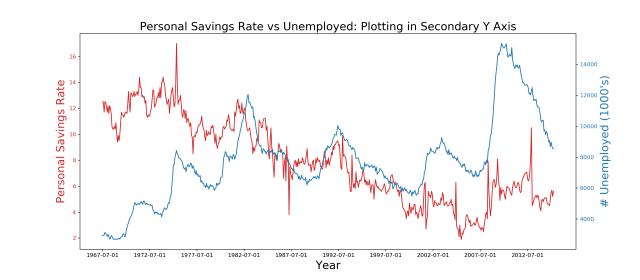## 14. Introduction to Seaborn

As the charts get more complex, the more the code you’ve got to write. For example, in matplotlib, there is no direct method to draw a density plot of a scatterplot with line of best fit. You get the idea.

So, what you can do instead is to use a higher level package like seaborn, and use one of its prebuilt functions to draw the plot.

We are not going in-depth into seaborn. But let’s see how to get started and where to find what you want. A lot of seaborn’s plots are suitable for data analysis and the library works seamlessly with pandas dataframes.

seaborn is typically imported as sns. Like matplotlib it comes with its own set of pre-built styles and palettes.

import seaborn as sns
sns.set_style("white")

# Import Data

# Draw Plot
plt.figure(figsize=(16,10), dpi= 80)
sns.kdeplot(df.loc[df['cyl'] == 4, "cty"], shade=True, color="g", label="Cyl=4", alpha=.7)
sns.kdeplot(df.loc[df['cyl'] == 6, "cty"], shade=True, color="dodgerblue", label="Cyl=6", alpha=.7)
sns.kdeplot(df.loc[df['cyl'] == 8, "cty"], shade=True, color="orange", label="Cyl=8", alpha=.7)

# Decoration
plt.title('Density Plot of City Mileage by n_Cylinders', fontsize=22)
plt.legend()
plt.show()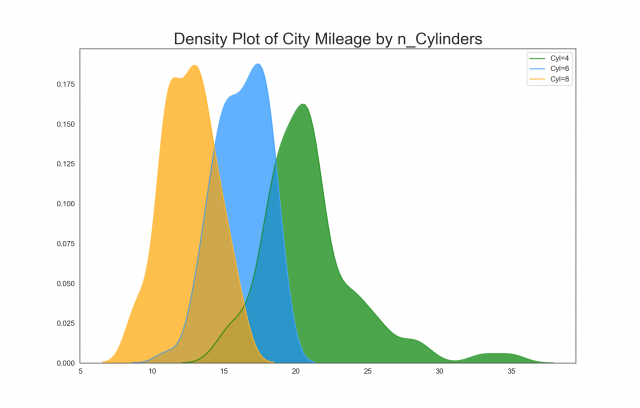# Load Dataset

# Plot
plt.figure(figsize=(10,8), dpi= 80)
sns.pairplot(df, kind="reg", hue="species")
plt.show()

<Figure size 800x640 with 0 Axes>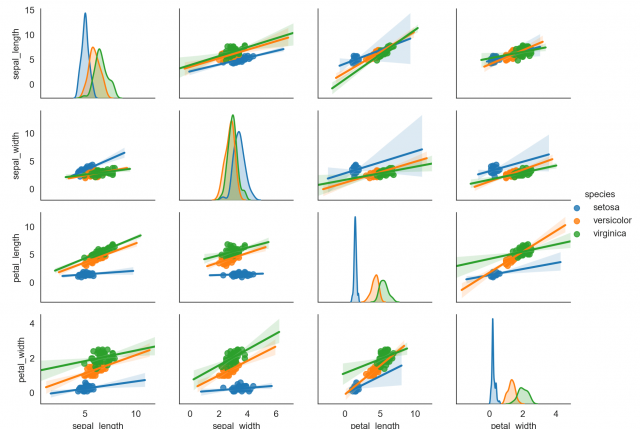This is just to give a hint of what’s possible with seaborn. Maybe I will write a separate post on it. However, the official seaborn page has good examples for you to start with.

## 15. Conclusion

Congratulations if you reached this far. Because we literally started from scratch and covered the essential topics to making matplotlib plots.

We covered the syntax and overall structure of creating matplotlib plots, saw how to modify various components of a plot, customized subplots layout, plots styling, colors, palettes, draw different plot types etc.

If you want to get more practice, try taking up couple of plots listed in the top 50 plots starting with correlation plots and try recreating it.

Until next time!